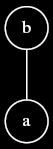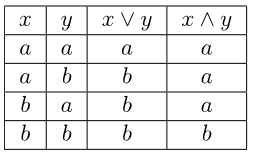# Lattice: Examples

Here are some additional examples of lattices. $$\newcommand{\nsubg}{\mathcal N \mbox{-} Sub~G}$$

## A familiar example

Consider the following lattice.Does this lattice look at all familiar to you? From some other area of mathematics, perhaps?

In fact, this lattice corresponds to boolean logic, as can be seen when we replace b with true and a with false in the following “truth table”.<div><div>comment:

Latex source:

\begin{tabular} {| c | c | c | c |} \hline $$x$$ & $$y$$ & $$x \vee y$$ & $$x \wedge y$$ \ \hline $$a$$ & $$a$$ & $$a$$ & $$a$$ \ \hline $$a$$ & $$b$$ & $$b$$ & $$a$$ \ \hline $$b$$ & $$a$$ & $$b$$ & $$a$$ \ \hline $$b$$ & $$b$$ & $$b$$ & $$b$$ \ \hline \end{tabular}

%%

%%

## Normal subgroups

Let $$G$$ be a group, and let $$\nsubg$$ be the set of all normal subgroups of $$G$$. Then $$\langle \nsubg, \subseteq \rangle$$ is a lattice where for $$H, K \in \nsubg$$, $$H \wedge K = H \cap K$$, and $$H \vee K = HK = \{ hk \mid h \in H, k \in K \}$$.

Let $$H,K \in \nsubg$$. Then $$H \wedge K = H \cap K$$. We first note that $$H \cap K$$ is a subgroup of $$G$$. For let $$a,b \in H \cap K$$. Since $$H$$ is a group, $$a \in H$$, and $$b \in H$$, we have $$ab \in H$$. Likewise, $$ab \in K$$. Combining these, we have $$ab \in H \cap K$$, and so $$H \cap K$$ is satisfies the closure requirement for subgroups. Since $$H$$ and $$K$$ are groups, $$a \in H$$, and $$a \in K$$, we have $$a^{-1} \in H$$ and $$a^{-1} \in K$$. Hence, $$a^{-1} \in H \cap K$$, and so $$H \cap K$$ satisfies the inverses requirement for subgroups. Since $$H$$ and $$K$$ are subgroups of $$G$$, we have $$e \in H$$ and $$e \in K$$. Hence, we have $$e \in H \cap K$$, and so $$H \cap K$$ satisfies the identity requirement for subgroups.

Furthermore, $$H \cap K$$ is a normal subgroup, because for all $$a \in G$$, $$a^{-1}(H \cap K)a = a^{-1}Ha \cap a^{-1}Ka = H \cap K$$. It’s clear from the definition of intersection that $$H$$ and $$K$$ do not share a common subset larger than $$H \cap K$$.

For $$H, K \in \nsubg$$, we have $$H \vee K = HK = \{ hk \mid h \in H, k \in K \}$$.

First we will show that $$HK$$ is a group. For $$hk, h'k' \in HK$$, since $$kH = Hk$$, there is some $$h'' \in H$$ such that $$kh' = h''k$$. Hence, $$hkh'k' = hh''kk' \in HK$$, and so $$HK$$ is closed under $$G$$’s group action. For $$hk \in HK$$, we have $$(hk)^{-1} = k^{-1}h^{-1} \in k^{-1}H = Hk^{-1} \subseteq HK$$, and so $$HK$$ is closed under inversion. Since $$e \in H$$ and $$e \in K$$, we have $$e = ee \in HK$$. Finally, $$HK$$ inherits its associativity from $$G$$.

To see that $$HK$$ is a normal subgroup of $$G$$, let $$a \in G$$. Then $$a^{-1}HKa = Ha^{-1}Ka = HKa^{-1}a = HK$$.

There is no subgroup $$F$$ of $$G$$ smaller than $$HK$$ which contains both $$H$$ and $$K$$. If there were such a subgroup, there would exist some $$h \in H$$ and some $$k \in K$$ such that $$hk \not\in F$$. But $$h \in F$$ and $$k \in F$$, and so from $$F$$’s group closure we conclude $$hk \in F$$, a contradiction.

<div><div>

Parents: